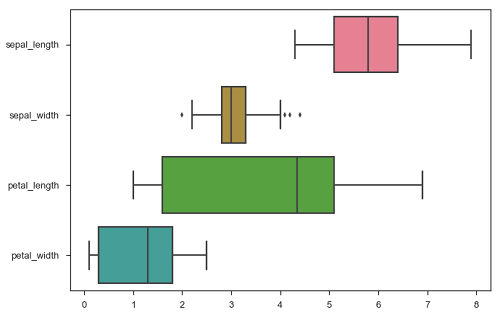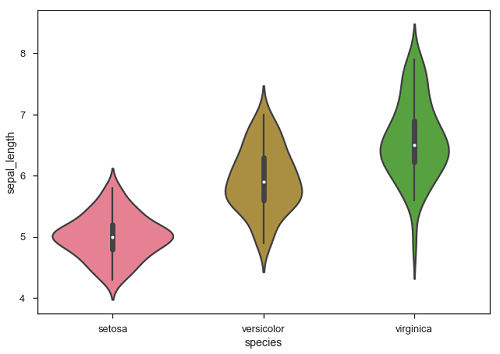# Seaborn - Plotting Wide Form Data

It is always preferable to use ‘long-from’ or ‘tidy’ datasets. But at times when we are left with no option rather than to use a ‘wide-form’ dataset, same functions can also be applied to “wide-form” data in a variety of formats, including Pandas Data Frames or two-dimensional NumPy arrays. These objects should be passed directly to the data parameter the x and y variables must be specified as strings

## Example

```import pandas as pd
import seaborn as sb
from matplotlib import pyplot as plt
sb.boxplot(data = df, orient = "h")
plt.show()
```

### OutputAdditionally, these functions accept vectors of Pandas or NumPy objects rather than variables in a DataFrame.

### Example

```import pandas as pd
import seaborn as sb
from matplotlib import pyplot as plt
sb.boxplot(data = df, orient = "h")
plt.show()
```

### OutputThe major advantage of using Seaborn for many developers in Python world is because it can take pandas DataFrame object as parameter.